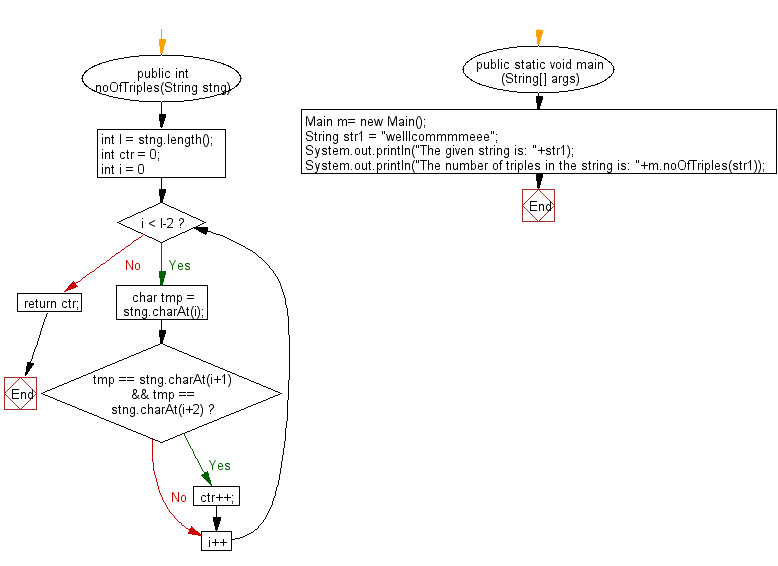﻿ Java exercises: Count the number of triples (characters appearing three times in a row) in a given string - w3resource# Java String Exercises: Count the number of triples (characters appearing three times in a row) in a given string

## Java String: Exercise-86 with Solution

Write a Java program to count the number of triples (characters appearing three times in a row) in a given string.

Sample Solution:

Java Code:

``````import java.util.*;
public class Main
{
public int noOfTriples(String stng)
{
int l = stng.length();
int ctr = 0;
for (int i = 0; i < l-2; i++)
{
char tmp = stng.charAt(i);
if (tmp == stng.charAt(i+1) && tmp == stng.charAt(i+2))
ctr++;
}
return ctr;
}

public static void main (String[] args)
{
Main m= new Main();
String str1 =  "welllcommmmeee";
System.out.println("The given string is: "+str1);
System.out.println("The number of triples in the string is: "+m.noOfTriples(str1));
}
}
``````

Sample Output:

```The given string is: welllcommmmeee
The number of triples in the string is: 4
```

Flowchart:Java Code Editor:

Improve this sample solution and post your code through Disqus

What is the difficulty level of this exercise?

﻿

## Java: Tips of the Day

Parsing dates:

```import java.io.*;
import java.util.*;
import java.text.*;

String s = "2001/09/23 14:39";

SimpleDateFormat formatter = new SimpleDateFormat ("yyyy/MM/dd H:mm");
Date d = formatter.parse(s, new ParsePosition(0));
```